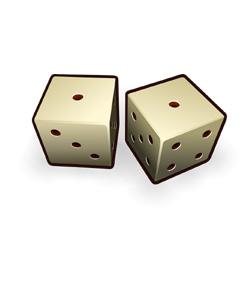# Probability (Math) Quiz

15 Questions | Total Attempts: 1963SettingsProbability in mathematics explores the concept, which requires broader thinking out of the box. Gauge your knowledge on probability and how far you can stretch your thoughts through the quiz below and learn more. Enjoy.

Related Topics
• 1.
A coin is tossed 1000 times and 560 times a ‘head’ occurs. The empirical probability of occurrence of a Head in this case is:
• A.

0.5

• B.

0.56

• C.

0.44

• D.

0.056

• 2.
Two coins are tossed 200 times and the following outcomes are recorded: What is the empirical probability of occurrence of at least one Head in the above case?
• A.

0.33

• B.

0.34

• C.

0.66

• D.

0.83

• 3.
A die is thrown 200 times and the following outcomes are noted, with their frequencies: What is the empirical probability of getting a 1 in the above case?
• A.

0.28

• B.

0.22

• C.

0.21

• D.

0.15

• 4.
A die is thrown 200 times and the following outcomes are noted, with their frequencies: What is the empirical probability of getting a number less than 4?
• A.

0.50

• B.

0.52

• C.

0.54

• D.

0.46

• 5.
A die is thrown 200 times and the following outcomes are noted, with their frequencies: What is the empirical probability of getting a number greater than 4?
• A.

0.18

• B.

0.25

• C.

0.32

• D.

0.46

• 6.
On a particular day, the number of vehicles passing a crossing is given below: What is the probability of a two wheeler passing the crossing on that day?
• A.

0.26

• B.

0.71

• C.

0.385

• D.

0.615

• 7.
The following table shows the blood-groups of 100 students: One student is taken at random. What is the probability that his blood group is B+?
• A.

0.35

• B.

0.20

• C.

0.12

• D.

0.10

• 8.
In a bag, there are 100 bulbs out of which 30 are bad ones. A bulb is taken out of the bag at random. The probability of the selected bulb to be good is:
• A.

0.30

• B.

0.50

• C.

0.70

• D.

None of these

• 9.
A bag contains 40 red, 30 blue and 30 green balls. A ball is drawn at random. What is the probability the ball drawn is a red ball?
• A.

0.30

• B.

0.40

• C.

0.60

• D.

0.70

• 10.
The king, queen and jack of clubs are removed from a deck of 52 playing cards and then the cards are well shuffled. A card is drawn at random from the rest of the cards. What is the probability that the card would be a King of clubs?
• A.

Zero

• B.

0.50

• C.

0.60

• D.

0.80

• 11.
An integer is chosen between 0 and 100. What is the probability that it is divisible by 9?
• A.

0.09

• B.

0.10

• C.

0.11

• D.

0.12

• 12.
Cards with number 1 to 100 are placed in a box. A card is selected at random. What is the probability that the card is a number divisible by 8?
• A.

0.10

• B.

0.11

• C.

0.09

• D.

0.12

• 13.
A letter of English alphabet is chosen at random. What is the probability that the letter is a vowel?
• A.

5/24

• B.

5/26

• C.

5/27

• D.

5/28

• 14.
There are 500 sealed envelopes in a box: If the envelopes are well shuffled and then an envelope is picked out randomly, what is the probability that it contains a no cash prize?
• A.

3/5

• B.

2/5

• C.

4/5

• D.

Zero

• 15.
A child’s game has 8 triangles of which 3 are blue and rest are red, and 10 squares of which 4 are blue and rest red. One piece is lost at random. What s the probability that it is a square of red colour?
• A.

2/9

• B.

2/5

• C.

3/5

• D.

1/3